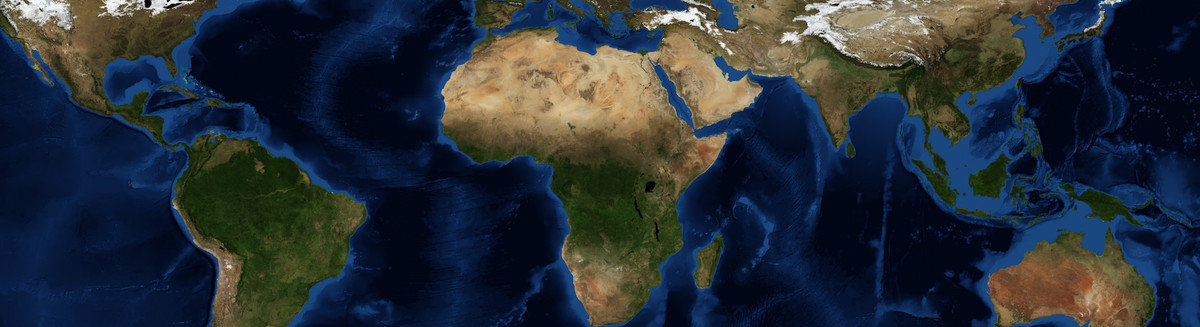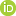This is a Preprint and has not been peer reviewed. The published version of this Preprint is available: https://doi.org/10.1029/2019JB017691. This is version 2 of this Preprint.

##### Authors

Guangdong Zhao, Bo Chen, Leonardo Uieda, Jianxin Liu, Mikhail K. Kaban, Longwei Chen, Rongwen Guo

##### Abstract

An efficient forward modeling algorithm for calculation of gravitational fields in spherical coordinates is developed for 3D large‐scale gravity inversion problems. 3D Gauss‐Legendre quadrature (GLQ) is used to calculate the gravitational fields of mass distributions discretized into tesseroids. Equivalence relations in the kernel matrix of the forward‐modeling are exploited to decrease storage and computation time. The numerical tests demonstrate that the computation time of the proposed algorithm is reduced by approximately two orders of magnitude, and the memory requirement is reduced by Nλ times compared with the traditional GLQ method, where Nλ is the number of the model elements in the longitudinal direction. These significant improvements in computational efficiency and storage make it possible to calculate and store the dense Jacobian matrix in 3D large‐scale gravity inversions. The equivalence relations can be applied to the Taylor series method or combined with the adaptive discretization to ensure high accuracy. To further illustrate the capability of the algorithm, we present a regional synthetic example. The inverted results show density distributions consistent with the actual model. The computation took about 6.3 hours and 0.88 GB of memory compared with about a dozen days and 245.86 GB for the traditional 3D GLQ method. Finally, the proposed algorithm is applied to the gravity field derived from the latest lunar gravity model GL1500E. 3D density distributions of the Imbrium and Serenitatis basins are obtained, and high‐density bodies are found at the depths 10‐60 km, likely indicating a significant uplift of the high‐density mantle beneath the two mascon basins.

##### DOI

https://doi.org/10.31223/osf.io/dzf9j

##### Subjects

Earth Sciences, Geophysics and Seismology, Physical Sciences and Mathematics

##### Keywords

Inversion, Moon, forward-modeling, gravity, tesseroid

##### Dates

Published: 2019-04-11 07:04